# Methods of Starting Electric Motor

## Methods of Starting Electric Motor:

Methods of Starting Electric Motor – Transient processes involved with the starting of the (drive) motor in a variable speed drive require a detailed study. The electric motor and connected load accelerates to the rated speed from rest under the influence of the starting torque. The transient operation during  is satisfactory if a sufficiently good starting torque is developed with a reduced value of starting current, to accelerate the rotor in the desired amount of time.

The need to limit the starting current arises due to heavy dips of the voltage of the motor following starting peaks of current. The starting equipment used should be capable of minimising these dips in the voltage to a tolerable value, so that the other equipment on the network is not affected.

The starting current affects the motor also. High starting currents heat up the rotor. If starting is frequent, the heating needs to be reduced or limited. In dc machines high starting currents produce sparking at the brushes. For good commutation the starting currents must be limited.

In converter operation additional harmonics of current affect the commuta­tion. These problems of commutation in dc motors due to ripple may be solved by (properly) increasing the pulse number of the power converter, and modifying the design of the motor itself, e.g. laminating the interpoles.

The starting torque must produce uniform acceleration. Acceleration time must be reduced with a view to improving the productivity and to reduce the energy lost during starting.

The purpose of starting equipment in an electric motor is to limit the starting current and to provide a reasonably good starting torque, if possible, so that the motor accelerates in the desired period to the rated speed. For dc motors the starting current is limited by using an additional resistance in series with the armature. The motor is switched on with full field. This is effectively reduced voltage starting. Thyristor power converters used for speed control may also be used for the purpose of starting, since the voltage is smoothly variable and starting losses are absent.

Induction motors are started by any of the following methods:

1. Direct on line starting
2. Low voltage starting
3. Rotor resistance starting
4. Low frequency starting
5. Special rotor construction.

A comparison of these methods is given in Table 1.6.

Synchronous motors are not self starting, but are started by an auxiliary motor or using the induction motor principle. The damper windings are used as starting windings. To make a synchronous motor self starting, a wound rotor is employed. It is short circuited with an additional resistance during starting and fed from a dc when accelerated to synchronous speed. A synchronous motor can also be started using a variable frequency inverter system. A comparison of variable frequency starting with mains starting is given in Fig. 1.52.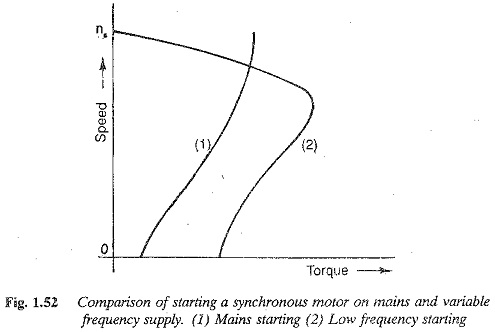### Energy Relations During Starting

In order to select a proper starting equipment, e.g. the starting rheostat in a shunt motor, it becomes necessary to determine the energy loss during starting.

1.The energy loss during starting is the K.E. of the rotating parts at fi­nal speed. The same energy is drawn from the supply. Therefore the total electrical energy drawn from the supply during starting is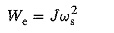where ωs is the final speed at no-load.

When started against a load of torque TL the total loss in the armature

where ωL is the speed with load TL and TLF is the energy loss due to the load torque and is given byIn the case of dc series motors the energy loss depends on R, and is given by2.In the case of an induction motor, an additional rotor resistance is em­ployed. The energy loss in the rotor resistance is the K.E. of the rotating parts. Part of the energy is dissipated in the stator resistance also. The total energy lost during starting is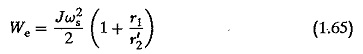An increase in rotor resistance decreases the energy loss in the stator resis­tance, whereas it does not affect the loss in the rotor resistance itself. This decreases the time of acceleration.

When the motor is started on load the loss dissipated is greater than at no-load, and is given bywhere Td and TL are functions of slip. The integration of ΔWSL from 0-ωs gives the total energy loss. When squirrel cage motors are started directly from the line, there is minimum energy dissipation because the torque developed is large with full voltage. With low voltage starting, Td decreases and the energy wasted increases, even though starting current decreases. This may be attributed to the increase of acceleration time at low voltage starting.

In squirrel cage motors the entire energy is lost in the machine itself, whereas in slip ring rotors an external resistance may be used for the dis­sipation of energy, thus minimising the motor heat but increasing the starting time.

### Starting Dynamics of Electric Motor

The starting of electric motor is normally done with graded resistances which are cut off slowly as the motor accelerates. The grading is based on two limits between which starting current is allowed to vary. The dynamics during starting may be necessary to actually find out the values of these resistances.

### DC shunt motor

When a shunt motor is acerlerated under load, the equations areUsing these relations we havewhere

ω0 is the no-load speed

ωL is the speed drop under load

Tm is the mechanical time constant.

If the motor is accelerated from ω1 to ω2 the solution of this equation may be obtained asIf the acceleration is from zero to ω0 we have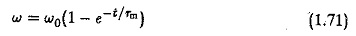During starting, the current drops as the motor speeds up, due to the building up of back emf. The armature current drops exponentially as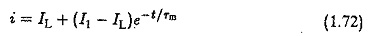where I1 is the starting current and IL the final value. If the acceleration is at no-load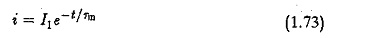The time of acceleration can be determined using these relations. If a multi-stepped starter is used, the time taken for the current to drop from I1 to I2 isThe mechanical time constant is different at different steps, and is given by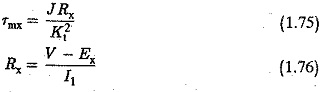The value of Ex is obtained by using the value of speed, which increases exponentially. Finally, the total accelerating time### Induction motor

We can analyse the transient conditions in a three-phase induction motor with a view to determining the time of acceleration. It is well known that by properly adjusting the rotor resistance, maximum torque may be made to occur at starting. But analysis shows that this does not give the minimum time of acceleration, which is obtained if the rotor resistance is selected such that the maximum torque occurs at a slip of 0.407.

The torque developed at any slip is given by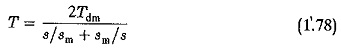Assuming the acceleration takes place at no-load, the torque developed accelerates the rotor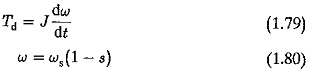Also, we have assuming the no-load speed is synchronous speed. Using the above equations we have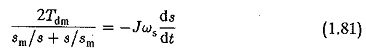form whichwhere Tm=Jωs∕Tdm is the mechanical time constant of the motor. The motor starts from rest and runs up to no-load speed. The slip varies from 1 to s. Integrating Eq. 1.82 between these limits we have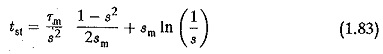If the final slip is assumed to be s = 0.03, the starting time tst isThe minimum value of tst is obtained by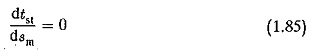which gives sm = 0.407. The ratio tst∕Tas a function of sm is shown in Fig. 1.51 To accelerate the rotor in the time tst the effective torque isThis shows again that the starting time is a minimum if the starting torque is 0.81 Tdm. This happens again if Tdm occurs at sm = 0.407. An induction motor can be made to accelerate in minimum time if the starting torque is 0.81 times the maximum torque and the maximum torque occurs at sm = 0.407.